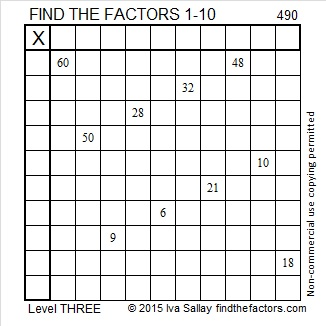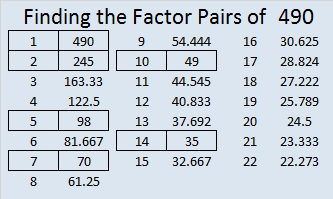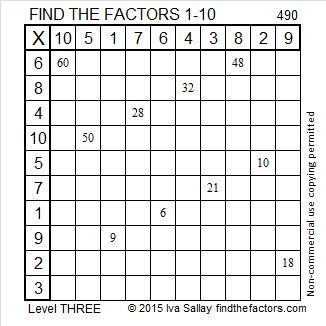# 490 and Level 3

490 is seventy times seven. A famous Bible verse mentioning this number is Matthew 18: 21 – 22.

This is the King James Version: “Then came Peter to him, and said, Lord, how oft shall my brother sin against me, and I forgive him? till seven times? Jesus saith unto him, I say not unto thee, Until seven times: but, Until seventy times seven.”

Most other Bible translation also have seventy times seven, but a few translations have only seventy seven. You can compare several different translations of Matthew 18: 22 here. Regardless of the translation, forgiveness is much more important than keeping count especially since all of us need to be forgiven as well.

To find √490 first notice that 490 can be evenly divided by 2, but not by 4. It also cannot be evenly divided by 9. It is divisible by 5, but since it doesn’t end in 25, 50, 75, or 00, it can only be divided by 5 once. Since it is divisible by 10, and dividing by 10 is so easy, I would make this one-layer cake:Then I would take the square root of everything on the outside of the cake and multiply together to get √490 = (√10)(√49) = 7√10.Print the puzzles or type the solution on this excel file: 10 Factors 2015-05-11

—————————————————————————————————

• 490 is a composite number.
• Prime factorization: 490 = 2 x 5 x 7 x 7, which can be written 490 = 2 x 5 x (7^2)
• The exponents in the prime factorization are 1, 1, and 2. Adding one to each and multiplying we get (1 + 1)(1 + 1)(2 + 1) = 2 x 2 x 3 = 12. Therefore 490 has exactly 12 factors.
• Factors of 490: 1, 2, 5, 7, 10, 14, 35, 49, 70, 98, 245, 490
• Factor pairs: 490 = 1 x 490, 2 x 245, 5 x 98, 7 x 70, 10 x 49, or 14 x 35
• Taking the factor pair with the largest square number factor, we get √490 = (√49)(√10) = 7√10 ≈ 22.135943—————————————————————————————————

A Logical Approach to solve a FIND THE FACTORS puzzle: Find the column or row with two clues and find their common factor. Write the corresponding factors in the factor column (1st column) and factor row (top row).  Because this is a level three puzzle, you have now written a factor at the top of the factor column. Continue to work from the top of the factor column to the bottom, finding factors and filling in the factor column and the factor row one cell at a time as you go.